I've set up a report using the table builder to send me daily any prices entered onto sales orders yesterday which are below the required profit margin. I'm using sales lines and then an ideal cost field from the item table.

I have added in a formula using to calculate the margin, I'm half way there in that the report is working, but I want to filter on these calculated values so I only get the prices that are too low rather than every sales line for the day.

Is there a way to do this? Please explain for a beginner as I've only been using for a few months and this is probably the most complex thing I've attempted!

•Harry Lewis

Hello Megan -

If you can re-state your comparison as an "unequal to" or "less than/greater than" question, this gets to be fairly simple.

I'm not saying it cannot be done differently, on that trying to build the function makes my head hurt.  ;-)

Here is an example (I have to draw from data examples for which I actually have data).

Let's suppose I'm drawing from a Dynamics NAV database (this particular technique relies on that).  I want records from the Sales Invoice Line where the Shipment Date does not match the Posting Date in the Sales Invoice Header table.

Note:  I could base this on any fields from any tables:  amount, quantity, etc.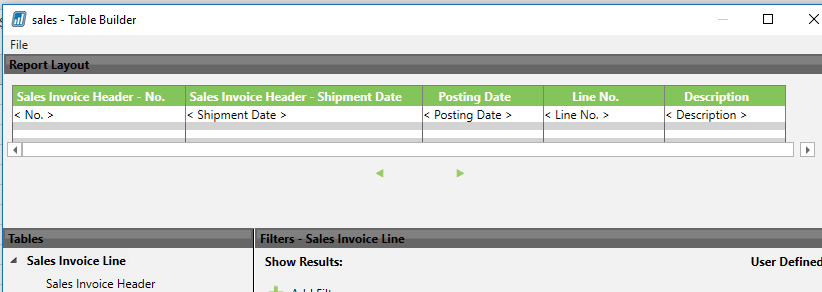For this particular case, I find it easier to add my data filtering in Excel (rather than the Table Builder).

Once I have my function: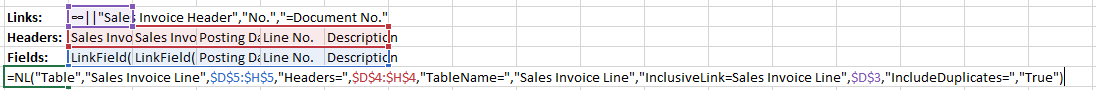I'll add the filter I need.  The FilterField will be the Shipment Date (here I've added that field and added a simple "*" for the filter):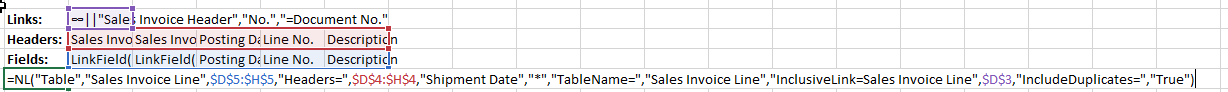Now, I'll add the filter for the Posting Date in the Sales Invoice Header: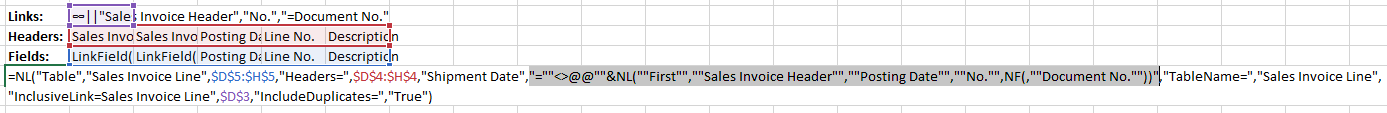What that filter says is "go out to the header get the first Posting Date where the No. field matches the Document No. field for the record I'm looking at in the Line table".

You could probably do some arithmetic by multiplying a value (e.g., a Cost field) by 0.7 or 1.3 in order to get more of a "percentage".

I hope that at least points you in the right direction.

•Megan F

Hi

Thank you for your response. Sorry I'm still confused to be honest, I can't work out how to apply your formula to what I want to do...think its a little too much brain power for me!

My report is below, I've already filtered by date to give me yesterday's date only. I then want to filter by margin which is a NP function formula, for values less than what ever I set (say <0.1 as an example), and it then give me every sales line that meets that criteria for that date. Is that even something that is possible?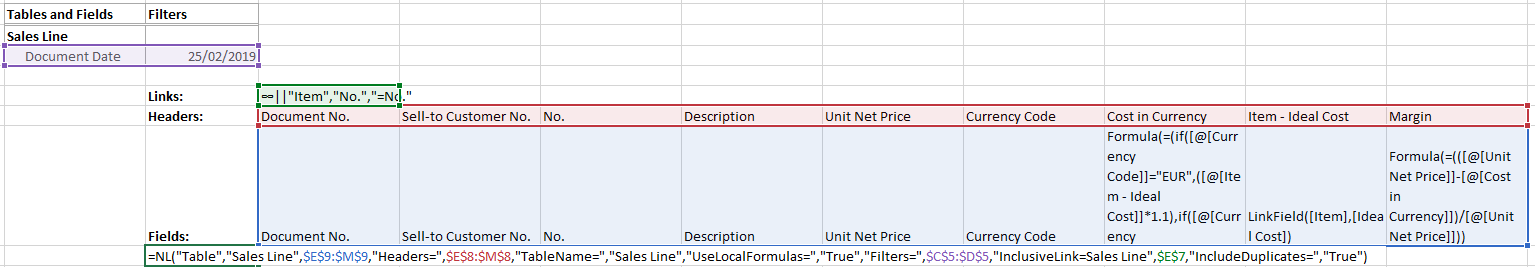•Harry Lewis

Hi Megan -

OK... here's what I tried.  (sorry for the long response, this is a rather advanced topic).

Since I cannot filter the NL("Table") function by a formula in the table, what I need to do is express that formula in terms of data in the database (rather than data in the table).

1) I don't have "Unit Net Price" or "Ideal Cost" fields.  So, I used the Unit Price from the Sales Line and the Unit Cost from the Item table.

2) I defined "Margin" as (Unit Price - Cost in Currency) / Unit Price

3) I assume that my NL(First) function is returning the same data as the Unit Cost in the NL(Table) function

4) I cannot read all of your "Cost In Currency" formula.  So I made up my own:

If Currency Code="EUR" then multiply Unit Cost by 0.9, otherwise multiply Unit Cost by 0.8

(keep in mind that I'm *not* saying that any of my formulae make sense... I just need some calculations to use for examples)

If I were to build this report using NL(Rows) function, instead of the NL(Table) function, it would look something like this: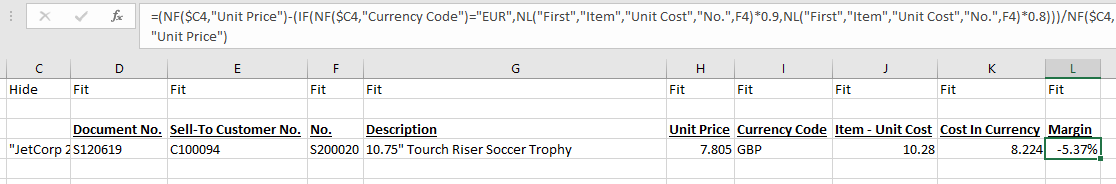I did this to illustrate that Margin formula.  As you can see, it is drawing data from the database.  I need to modify that function and use it to filter my NL("Table") function.

Speaking of which, here is what Table Builder created: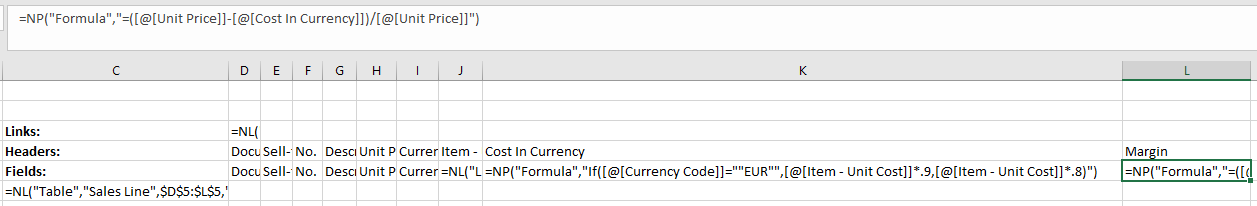As you can see, the Cost in Currency column and the Margin column are based on values in the table.  Again, we want that... but I can't FILTER on those.

Here is the NL(Table) function:

Let's simplify it just for illustration:

`=NL("Table","Sales Line",\$D\$5:\$L\$5,"Headers=",\$D\$4:\$L\$4,"InclusiveLink=Sales Line",\$D\$3)`

What I need to do is modify the Margin calculation that draws data from the database and then use the to filter the NL(Table) function/

I would refer to the Sort by Sum for NAV article for how to modify a calculation so it can be used as a Jet function filter.

That would allow me to take my calculation:

=(NF(\$C4,"Unit Price")-(IF(NF(\$C4,"Currency Code")="EUR",NL("First","Item","Unit Cost","No.",F4)*0.9,NL("First","Item","Unit Cost","No.",F4)*0.8)))/NF(\$C4,"Unit Price")

and turn it into this:

"=(NF(,""Unit Price"")-(IF(NF(,""Currency Code"")=""EUR"",NL(""First"",""Item"",""Unit Cost"",""No."",NF(,""No.""))*0.9,NL(""First"",""Item"",""Unit Cost"",""No."",NF(,""No.""))*0.8)))/NF(,""Unit Price"")"

Then, I have to add that as a FilterField to my NL(Table) function.  For arguments sake, let's assume I want to see data where that calculation is less than zero.

Here is the resulting NL(Table) function:

or... all in one line:

`=NL("Table","Sales Line",\$D\$5:\$L\$5,"Headers=",\$D\$4:\$L\$4,"=(NF(,""Unit Price"")-(IF(NF(,""Currency Code"")=""EUR"",NL(""First"",""Item"",""Unit Cost"",""No."",NF(,""No.""))*0.9,NL(""First"",""Item"",""Unit Cost"",""No."",NF(,""No.""))*0.8)))/NF(,""Unit Price"")","NUMBER&<0","InclusiveLink=Sales Line",\$D\$3)`

When I run my report...

Only those records where the Margin calculation is less than zero are returned: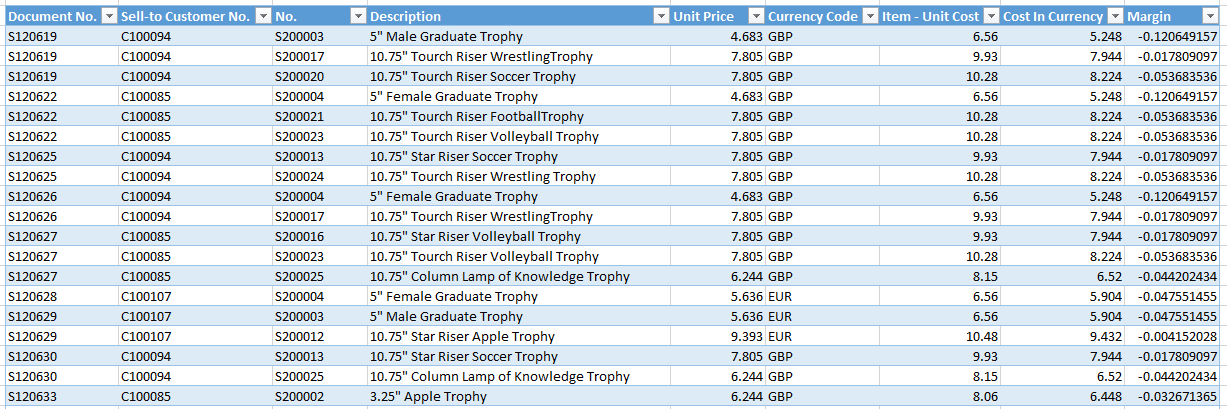As I stated at the beginning... this is an advanced technique.  The easiest way to address this is to simply use the NL(Table) function to dump all data and then use Excel's Pivot capabilities to show what you really want (or to use other Jet functions - e.g., NL(Rows) - so that you have greater control).

I hope that helps a little.

Edited by Harry Lewis